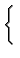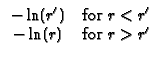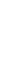R00 Infinite body, 0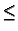r <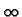. Note that this geometry requires a pseudo GF, denoted H. The temperature solution found from a pseudo GF requires that the total volumetric heating sums to zero and the spatial average temperature in the body must be supplied as a known condition.
2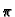HR00(r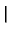r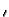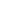) =